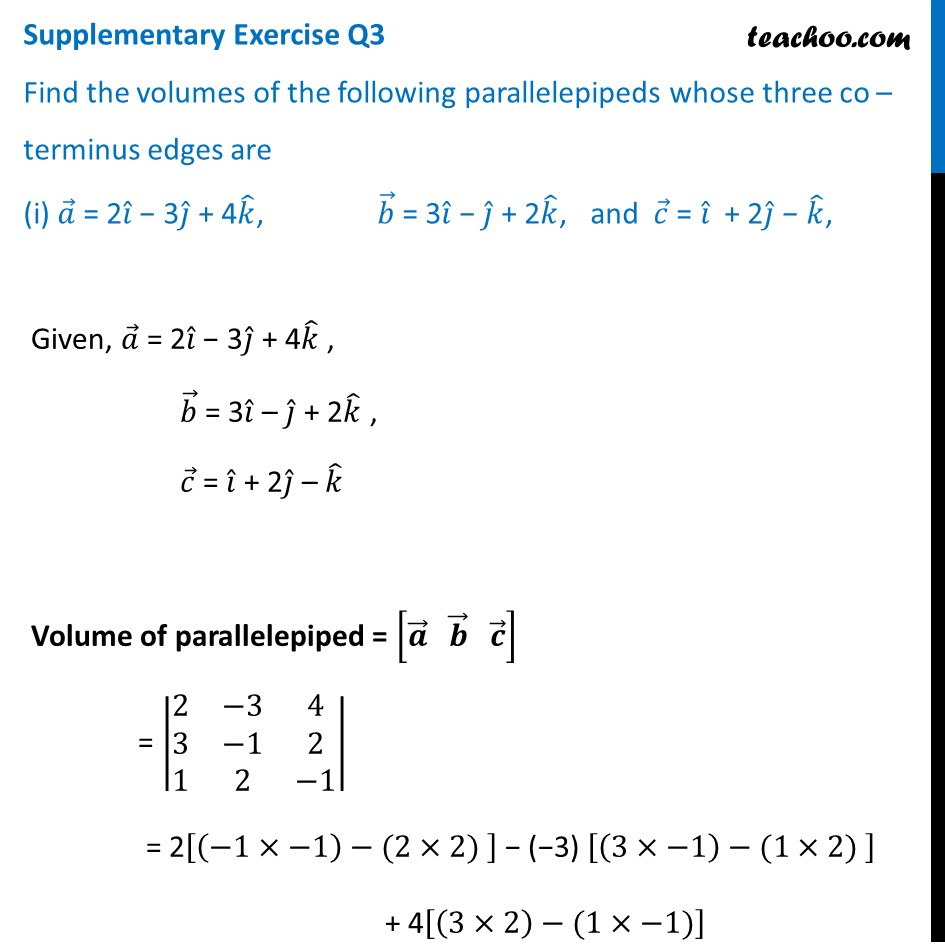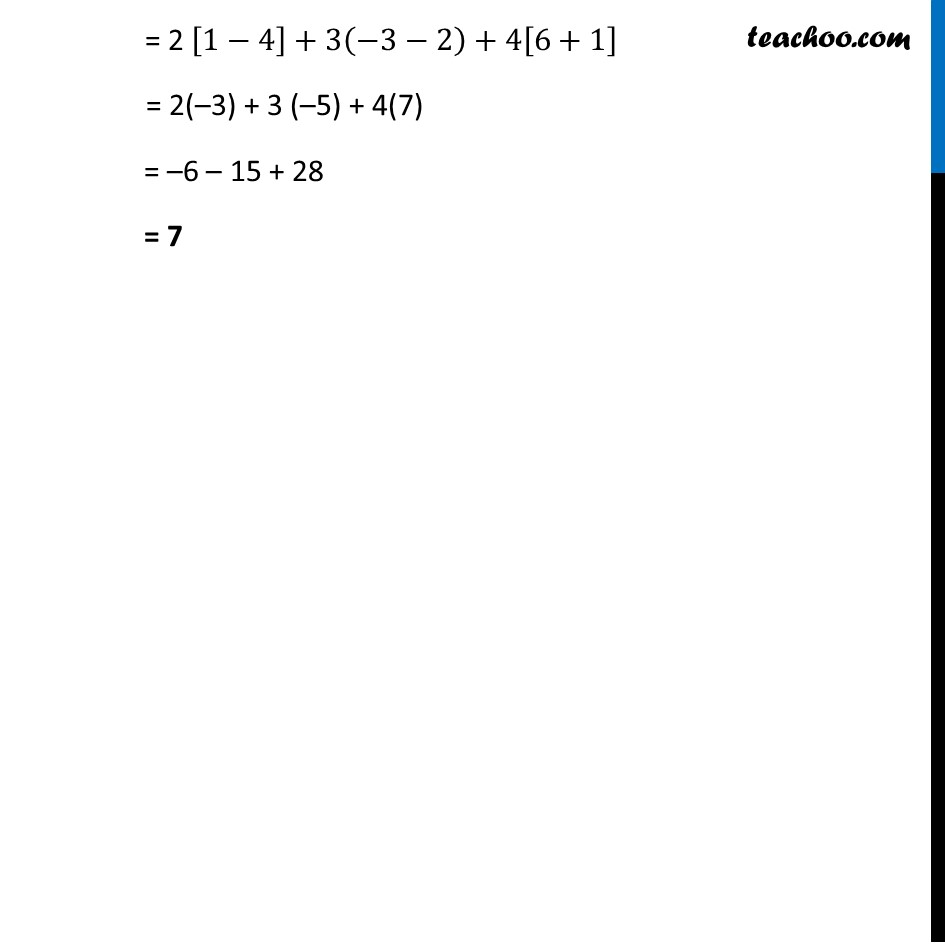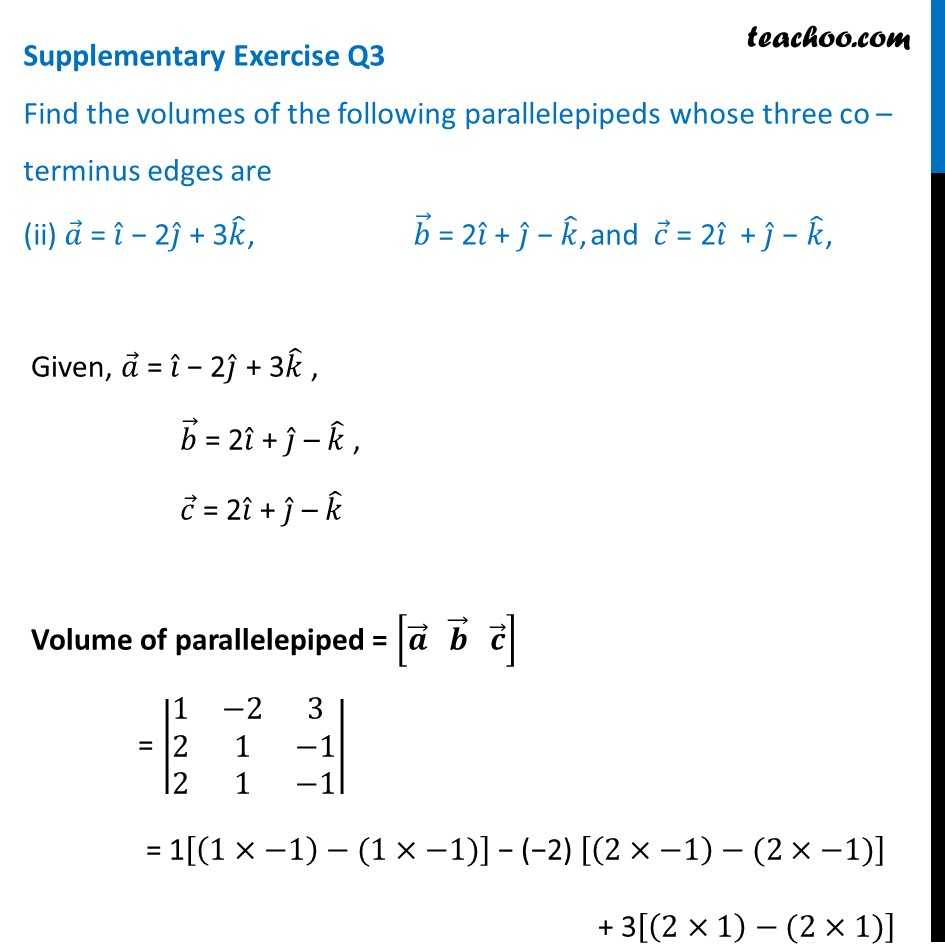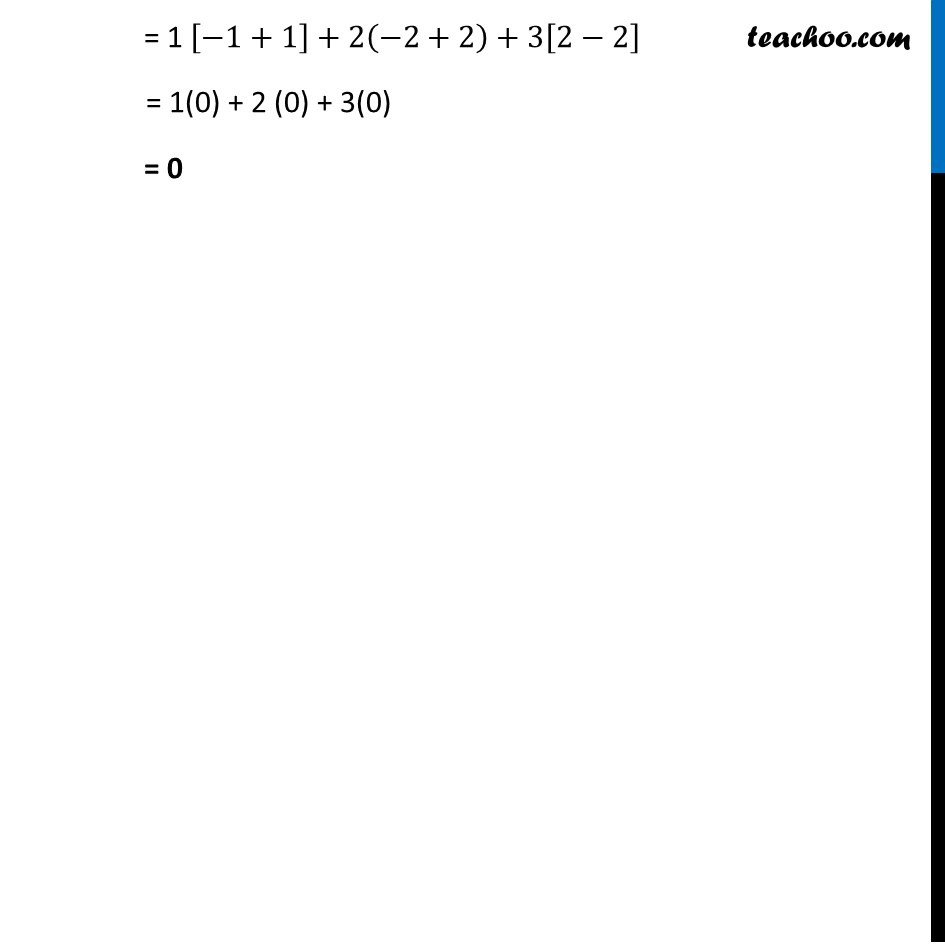Supplementary examples and questions from CBSE

Chapter 10 Class 12 Vector Algebra
Serial order wiseGet live Maths 1-on-1 Classs - Class 6 to 12

### Transcript

Supplementary Exercise Q3 Find the volumes of the following parallelepipeds whose three co –terminus edges are (i) 𝑎 ⃗ = 2𝑖 ̂ − 3𝑗 ̂ + 4𝑘 ̂, 𝑏 ⃗ = 3𝑖 ̂ − 𝑗 ̂ + 2𝑘 ̂, and 𝑐 ⃗ = 𝑖 ̂ + 2𝑗 ̂ − 𝑘 ̂, Given, 𝑎 ⃗ = 2𝑖 ̂ − 3𝑗 ̂ + 4𝑘 ̂ , 𝑏 ⃗ = 3𝑖 ̂ – 𝑗 ̂ + 2𝑘 ̂ , 𝑐 ⃗ = 𝑖 ̂ + 2𝑗 ̂ – 𝑘 ̂ Volume of parallelepiped = [𝒂 ⃗" " 𝒃 ⃗" " 𝒄 ⃗ ] = |■8(2&−3&[email protected]&−1&[email protected]&2&−1)| = 2[(−1×−1)−(2×2) ] − (−3) [(3×−1)−(1×2) ] + 4[(3×2)−(1×−1)] = 2 [1−4]+3(−3−2)+4[6+1] = 2(–3) + 3 (–5) + 4(7) = –6 – 15 + 28 = 7 Supplementary Exercise Q3 Find the volumes of the following parallelepipeds whose three co –terminus edges are (ii) 𝑎 ⃗ = 𝑖 ̂ − 2𝑗 ̂ + 3𝑘 ̂, 𝑏 ⃗ = 2𝑖 ̂ + 𝑗 ̂ − 𝑘 ̂, and 𝑐 ⃗ = 2𝑖 ̂ + 𝑗 ̂ − 𝑘 ̂, Given, 𝑎 ⃗ = 𝑖 ̂ − 2𝑗 ̂ + 3𝑘 ̂ , 𝑏 ⃗ = 2𝑖 ̂ + 𝑗 ̂ – 𝑘 ̂ , 𝑐 ⃗ = 2𝑖 ̂ + 𝑗 ̂ – 𝑘 ̂ Volume of parallelepiped = [𝒂 ⃗" " 𝒃 ⃗" " 𝒄 ⃗ ] = |■8(1&−2&[email protected]&1&−[email protected]&1&−1)| = 1[(1×−1)−(1×−1)] − (−2) [(2×−1)−(2×−1)] + 3[(2×1)−(2×1)] = 1 [−1+1]+2(−2+2)+3[2−2] = 1(0) + 2 (0) + 3(0) = 0